## plotting in sf compared to sp

Existing code, vignettes and materials relying on sp for plotting, subsetting, and user-chosen aesthetics linked to feature-data require modifications to provide analogous results. I feel that the current plotting code is too different to the sp-idioms, and I see value in maintaining what was a simple and powerful workflows that almost 100% consistent with base graphics. If the “relation_to_geometry” code stays in sf core then a new like ‘st_plot’ would allow compatibility with very common sp-expectations, and the pattern of sf functions now.

• packages relying of sf-core that don’t want the “relation_to_geometry” can follow the existing sp-idioms

Plot example

Currently to port this ‘sp’ code to use ‘sf’ requires significant changes that affect downstream code using these objects and workflows.

library(sp)
plot(x)
points(df$lon, df$lat)

plot(subset(x, acolumn == "countryname"), add = TRUE, col = "dodgerblue")


In sf to get this we need to do this

library(sf)
plot(st_geometry(x))
points(df$lon, df$lat)

plot(st_geometry(subset(x, acolumn == "countryname")), add = TRUE, col = "dodgerblue")


or this which requires now juggling two objects, and intermingling data.frame value logic with geometry logic separated into

library(sf)
gx <- st_geometry(x)
plot(gx)
points(df$lon, df$lat)

plot(gx[x$acolumn == 'countryname'], col = "dodgerblue") I think it’s important to make the transition from sp to st for existing users and package developers simpler, like this but currently this code will behave differently depending on what data columns are present, and require different strategies for use - all specific to a particular data set. library(sf) x <- st_read('dsn', 'layer') plot(x) points(df$lon, df$lat) plot(subset(x, acolumn == "countryname"), add = TRUE, col = "dodgerblue") It could be tricky to unpick some of the inter-related tasks that happen around geometry and length / area metrics, but I don’t see them as unsolvable and I’d commit significant effort into the design and development required to achieve this. ## Real-world code mgration example I took an example from the first result I found searching for “R plot wrld_simpl”. http://remi-daigle.github.io/GIS_mapping_in_R/ The code in one chunk is as follows, adapted to reduce duplication, and to use ‘wrld_simpl’ throughout rather than a local shapefile. library(maptools) ## Loading required package: sp ## Checking rgeos availability: TRUE data(wrld_simpl) plot(wrld_simpl)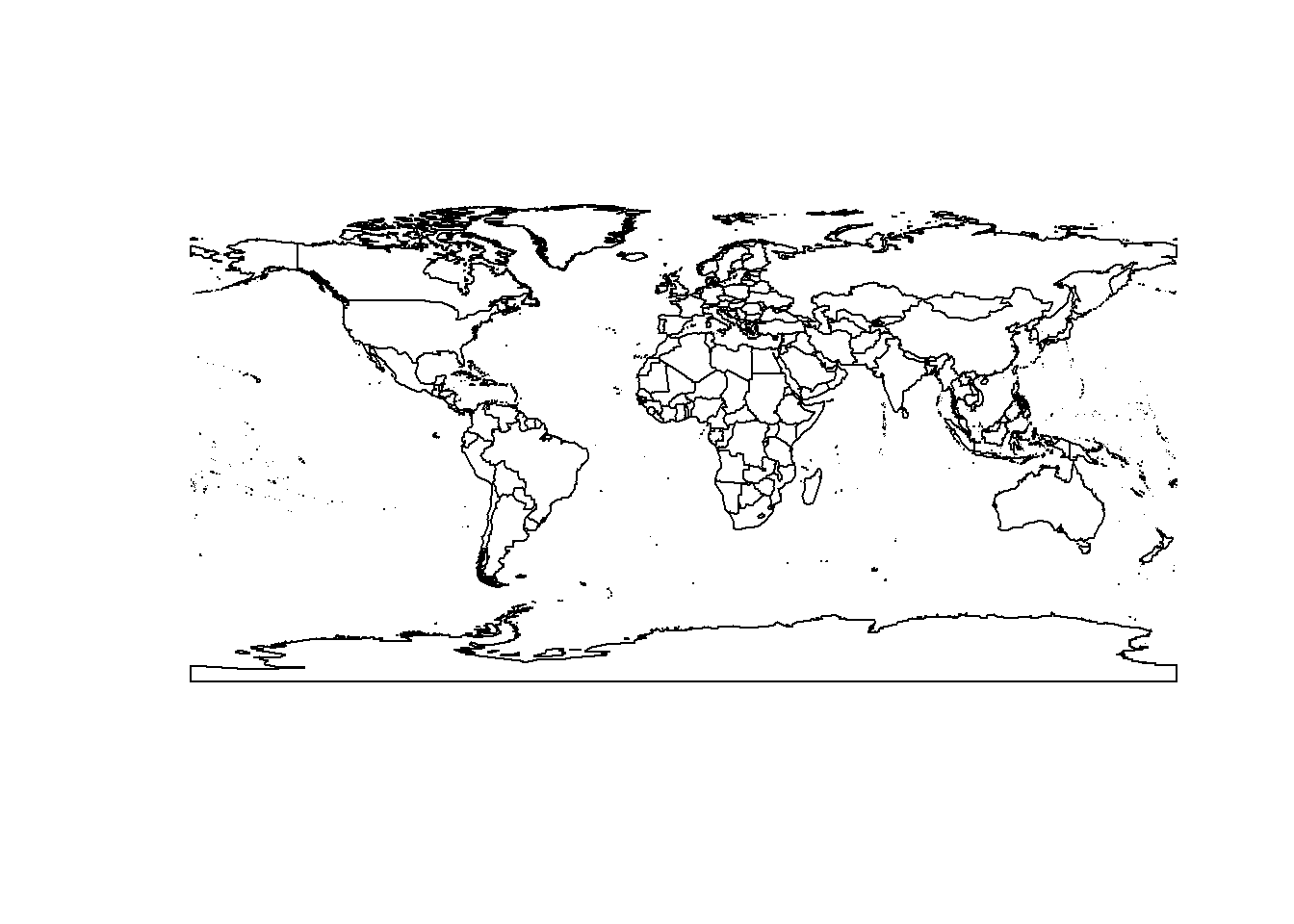xlim=c(-130,-60) ylim=c(45,80) plot(wrld_simpl,xlim=xlim,ylim=ylim)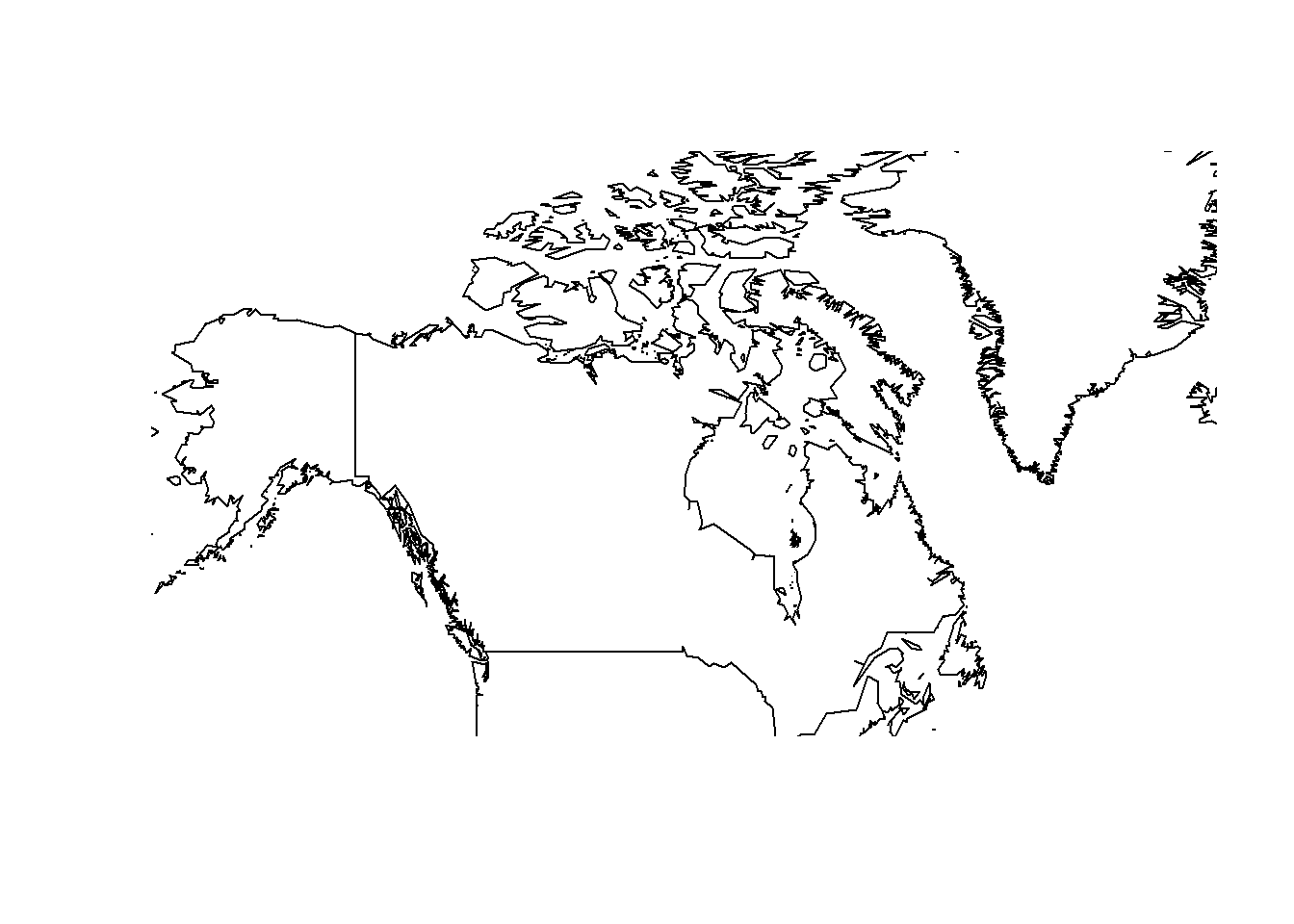plot(wrld_simpl,xlim=xlim,ylim=ylim,col='olivedrab3',bg='lightblue') ##world_shp <- readOGR(dsn = getwd(),layer = "world_test") ##plot(world_shp) coords <- matrix(c(-122.92,-79.4, 49.277,43.66),ncol=2) coords <- coordinates(coords) spoints <- SpatialPoints(coords) df <- data.frame(location=c("SFU","UofT")) spointsdf <- SpatialPointsDataFrame(spoints,df) plot(spointsdf,add=T,col=c('red','blue'),pch=16) coords <- matrix(c(-110,-102,-102,-110,-110,60,60,49,49,60),ncol=2) l <- Line(coords) ls <- Lines(list(l),ID="1") sls <- SpatialLines(list(ls)) df <- data.frame(province="Saskatchewan") sldf <- SpatialLinesDataFrame(sls,df) plot(sldf,add=T,col='black') #coords <- matrix(c(-110,-102,-102,-110,-110,60,60,49,49,60),ncol=2) p <- Polygon(coords) ps <- Polygons(list(p),ID="1") sps <- SpatialPolygons(list(ps)) df <- data.frame(province="Saskatchewan") spdf <- SpatialPolygonsDataFrame(sps,df) plot(spdf,add=T,col='red')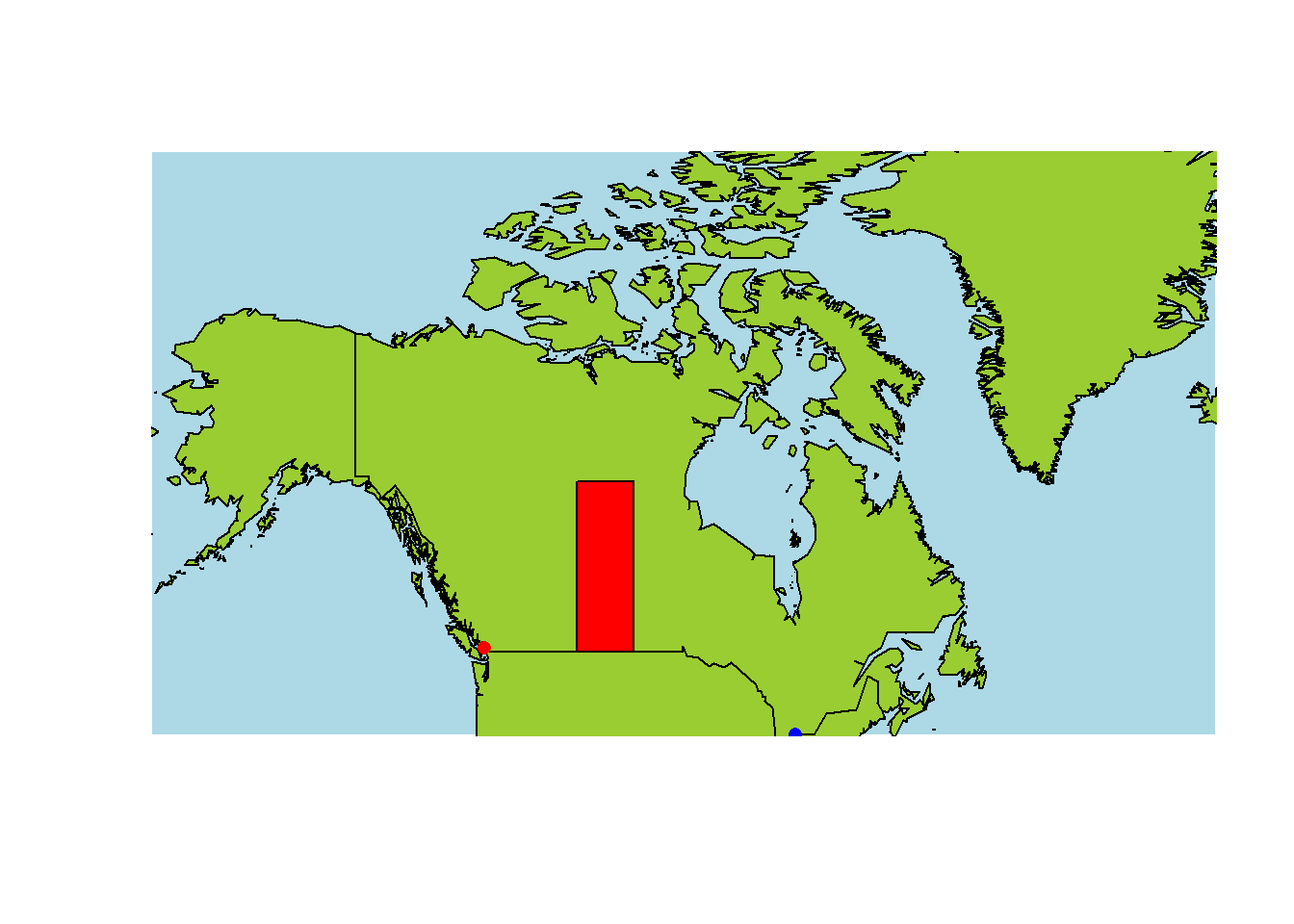# Canada <- getData('GADM', country="CAN", level=1) # plot(Canada) # NS <- Canada[Canada$NAME_1=="Nova Scotia",]
# plot(NS,col="blue")
#
# NB <- Canada[Canada$NAME_1=="New Brunswick",] # plot(NB,col="yellow",add=TRUE) # # PEI <- Canada[Canada$NAME_1=="Prince Edward Island",]
# plot(PEI,col="red",add=TRUE)

To get the same result I have to make several choices that are specific to this data set, which means that a lot of existing code will require bespoke modifications to migrate to sf.

First, what do I get if I make minimal changes that really are data structure specific? The original code was not ideal and an experienced sp user may have made many different choices, but it’s clear that the new construction tools make migration to sf an extremely very pleasant experience.

data("wrld_simpl", package = "maptools")
library(sf)
## Linking to GEOS 3.5.0, GDAL 2.1.1
wrld_simpl <- st_as_sf(wrld_simpl)
#plot(wrld_simpl)
#xlim=c(-130,-60)
#ylim=c(45,80)
#plot(wrld_simpl,xlim=xlim,ylim=ylim)
#plot(wrld_simpl,xlim=xlim,ylim=ylim,col='olivedrab3',bg='lightblue')
##world_shp <- readOGR(dsn = getwd(),layer = "world_test")
##plot(world_shp)
## much simpler than before!
spointsdf <- st_as_sf(data.frame(location=c("SFU","UofT"), lon = c(-122.92,-79.4), lat = c(49.277,43.66)),
coords = c("lon", "lat"), crs = 4326)
#coords <- coordinates(coords)
#spoints <- SpatialPoints(coords)
#df <- data.frame(location=c("SFU","UofT"))
#spointsdf <- SpatialPointsDataFrame(spoints,df)
#plot(wrld_simpl)
coords <- matrix(c(-110,-102,-102,-110,-110,60,60,49,49,60),ncol=2)
#l <- Line(coords)
#ls <- Lines(list(l),ID="1")
#sls <- SpatialLines(list(ls))
#sldf <- SpatialLinesDataFrame(sls,df)
sldf <- st_sf(geometry = st_sfc(st_linestring(coords), crs = 4326), province = "Saskatchewan")
#coords <- matrix(c(-110,-102,-102,-110,-110,60,60,49,49,60),ncol=2)
#p <- Polygon(coords)
#ps <- Polygons(list(p),ID="1")
#sps <- SpatialPolygons(list(ps))
#spdf <- SpatialPolygonsDataFrame(sps,df)
spdf <- st_sf(geometry = st_sfc(st_polygon(list(coords)), crs = 4326), province = "Saskatchewan")
#plot(spdf,add=T,col='red')  

In sf 0.2-7 the plotting is exactly the same as for sp and so no more work is required. With the current code in sf trunk we need completely different code. I think this is a problem for encouraging migration to sf, since the first steps are extremely pleasant, but then producing plots to prove that migration was successful requires a lot more work.

plot(wrld_simpl)xlim=c(-130,-60)
ylim=c(45,80)
plot(wrld_simpl,xlim=xlim,ylim=ylim)plot(wrld_simpl,xlim=xlim,ylim=ylim,col='olivedrab3',bg='lightblue')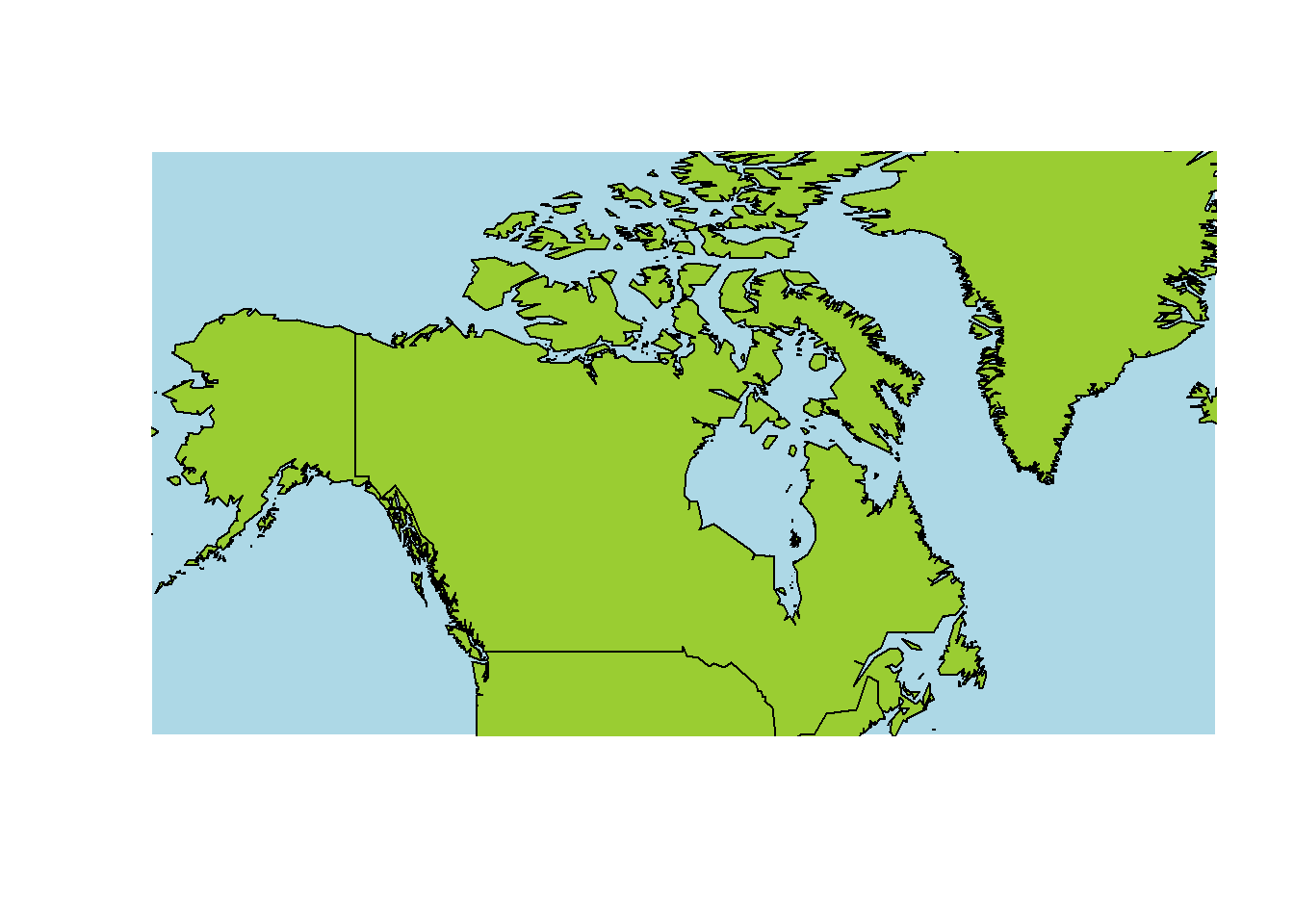plot(wrld_simpl)

plot(sldf,add=T,col='black')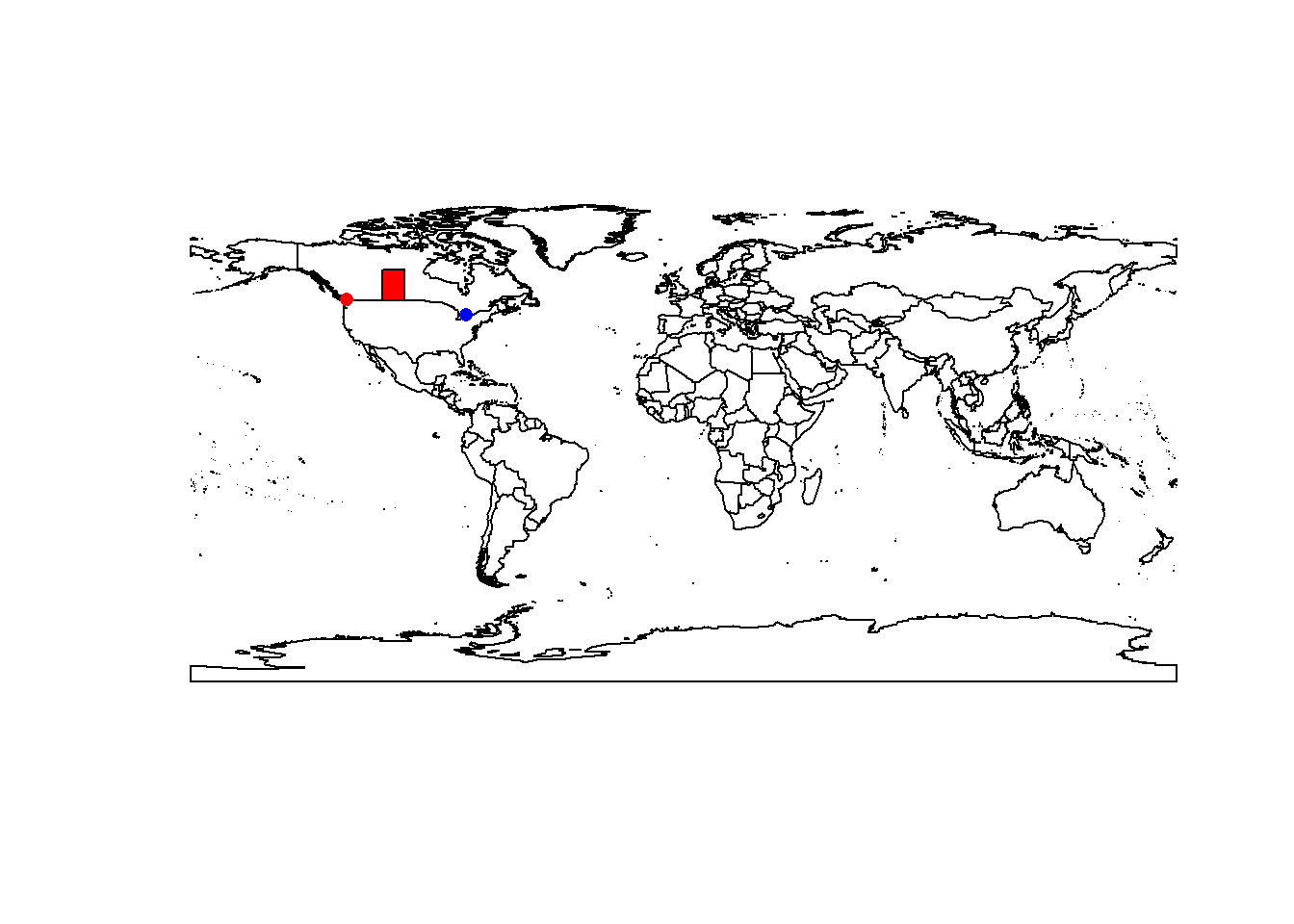## Document build details

sessionInfo()
## R version 3.3.2 Patched (2017-01-13 r71966)
## Platform: x86_64-w64-mingw32/x64 (64-bit)
## Running under: Windows 7 x64 (build 7601) Service Pack 1
##
## locale:
##  LC_COLLATE=English_Australia.1252  LC_CTYPE=English_Australia.1252
##  LC_MONETARY=English_Australia.1252 LC_NUMERIC=C
##  LC_TIME=English_Australia.1252
##
## attached base packages:
##  stats     graphics  grDevices utils     datasets  methods   base
##
## other attached packages:
##  sf_0.2-7        maptools_0.8-41 sp_1.2-4
##
## loaded via a namespace (and not attached):
##   Rcpp_0.12.9     lattice_0.20-34 digest_0.6.11   rprojroot_1.1
##   grid_3.3.2      DBI_0.5-1       backports_1.0.4 magrittr_1.5
##   evaluate_0.10   stringi_1.1.2   rmarkdown_1.3   rgdal_1.2-5
##  tools_3.3.2     stringr_1.1.0   foreign_0.8-67  yaml_2.1.14
##  htmltools_0.3.5 knitr_1.15.1